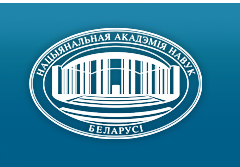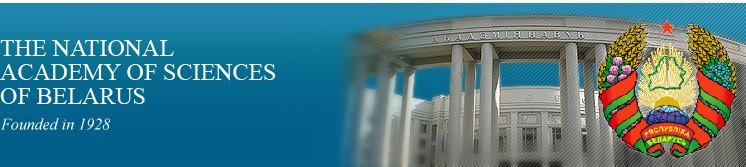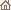Home News About Academy Academy Activities Academy Membership Organizations Publications Conferences Contacts Site Map

# Proc. of the Natl. Academy of Sciences of Belarus, Ser. Phys.-Math. Sci., 2000, No.1/ Publications / Scientific Journals

Proc. of the Natl. Academy of Sciences of Belarus, Ser. Phys.-Math. Sci., 2000, No.1Proceedings of the National Academy of Sciences of Belarus (Vestsi Natsiyanalnai Akademii Navuk Belarusi) SERIES OF PHYSICAL-MATHEMATICAL SCIENCESPublished by The Institute of Mathematics of NASB, Minsk, Republic of Belarus

### Number 1, 2000## CONTENTS

### MATHEMATICS

Gulo I.N., Yanovich L.A.
Laplace's method for one class of functional integrals. pp. 5--11

Summary: The asymptotic estimations for simple and multiple functional integrals by Laplace method are constructed.

Tyutyanov V.N.
Dp-theorem for finite groups having composition factors such that 2-length of any solvable semigroup do not exceed one. pp. 12--14.

Summary: New class of finite groups are found. Dp -theorem fulfill for this class.

Malyutin V.B.
Approximation of functional integrals with respect to space of functions taking values in space of odd elements of Grassman algebra. pp. 15--17

Summary: Approximate formulas for functional integrals on space of functions taking values in L1 are constructed.

Zherelo A.V.
Approximation of integrals with respect to measures, generated by solutions of stochastic differential equations on Poisson process. pp. 18--22

Summary: Approximate formulas having the given approximate degree of accuracy for functional integrals with respect measures generated by the solutions of differential stochastic equations on centered Poisson process and stochastic centered Poisson measure are obtained.

Kovalevskaya E.I.
On the exact order of simultaneous approximations for almost all linear manifold points. pp. 23--27

Summary: An analogue of Khinchine's theorem (the case of the convergence) is proved for linear manifolds points in the form (t, a1t,. . ., ant) where a1,. . . , an are badly approximable numbers.

Baulin A.S., Kalitin B.S.
On Lyapunov's functions of constant signs method for nonautonomous discrete systems. pp. 28--34

Summary: The second Lyapunov's method theorems for discrete systems are considered.

Beresnevich V.V.
Application of the concept of regular systems of points in metric number theory. pp. 35--39

Summary: The best regular systems are shown to result in the proof of Khintchine type theorem.

Kostyukova O.I.
Study of dependence of solutions to linear-quadratic optimal control problems on an initial state vector. pp. 40--45

Summary: A family of linear-quadratic optimal control problems is considered. Properties of solutions and performance index function are studied. A special attention is paid to nonregular situations.

Bruj I.N.
Trigonometric series of classes Lp(T) and their matrix-means. pp. 46--49

Summary:Tsynkevich N.A.
On the conditions of asymptotic stability based on the estimate of the right-hand side. pp. 50--55

Summary: The generalization of the asymptotic stability condition for the zero solution of nonautonomous differential equation in the case of small estimate of the derivative of auxiliary function is proved.

Metelskii N.N., Naidenko V.G.
On a class of partially convex semispaces. pp. 56--59

Summary: A characterization of the conic semispaces of partial convexity is obtained. The number of these semispaces is estimated for a finite directions set. A polynomial rate algorithm for enumerating the conic semispaces is developed.

Meleshko I.N., Lasy P.G.
On approximation of functions by polylogarithms. pp. 60--64

Summary: New formulas for approximate calculation of functions which are differentiable on a segment are given. The proposed formulas use polylogarithms.

Benediktovich V.I., Konovalov O.L., Sarvanov V.I.
Construction of a body in regular polyhedron in R3. pp. 65--69

Summary: The questions connected with the finding of (minimum) closed bodies of finite volums in regular polyhedron in R3 are regarded. The algorithms of complexity O(n3/ log n) for their solutions are proposed.

Lepin V.V.
A local search algorithm for the hypergraph linear arrangements problem. pp. 70--74

Summary: A new local search algorithm for solving the hypergraph linear arrangements problem is developed. It is proved that finding a local optimum for this problem, with the neighborhood structure defined in the algorithm, is PLS-complete.

### PHYSICS

Komarov F.F., Leont'ev A.V., Grigor'ev V.V., Gran'ko S.V.
Ranges of boron ions in organic materials. pp. 75--80

Summary: The experimental results of boron ion ranges in polyimide and PMMA are presented. The comparison of obtained data was carried out relative to calculated results fulfilled by Monte-Carlo method and data depending on trajectory parameters of boron ions in different polymers.

Revinskii A.F., Chichurin A.V.
About one method of solution of the soliton equation for the interacting phonon gas. pp. 81--84

Summary: The higher order nonlinearity Korteweg-de Vries (KdV) equation for a one-dimensional anharmonic lattice is described. The generalized solutions of KdV equation are obtained.

Kapshai V.N., Fursin A.I.
Consequences of the system of gauge and field equations in the gauge dµAaµ + fa = 0 for the non-Abelian fields. pp. 85--89

Summary: The equation (with the help of mathematical apparatus of functional integration) as a consequence of the system of gauge and field equations in the gauge dµAaµ + fa = 0 is obtained. This equation was checked with respect to the lowest order of the perturbation theory.

Red'kov V.M.
On equations for the Dirac--Kahler field and bosons with different intrinsic parities in the Riemann space-time. pp. 90--95

Summary: The article relates a generally covariant tensor formalism to a spinor one when these both are applied to description of the Dirac--Kahler field in the Riemann space-time. Both methods are taken to be equivalent and the tensor equations are derived from spinor ones. It is shown that, for characterization of Dirac--Kahler's tensor components, two alternative approaches are suitable: these are whether a tetrad-based pseudo tensor classification or a generally coordinate pseudo tensor one. By imposing definite restricting conditions on the the Dirac--Kahler function, we have produced the general covariant form of wave equations (both in tensor and spinor form) for scalar, pseudoscalai, vector, and pseudovector particles.

Novysh B.V., Dorozhkin N.N.
Algorithm for calculation of Cooper pairs wave functions for anisotropic pairing potentials. pp. 96--98

Summary: Calculation scheme to solve Cooper pair problem is presented using the expansion of amplitudes and pairing potential into angular components. The scheme may be used in the process of self-consistent solution of BCS-type system of equations for anisotropic pairing potentials.

Tochitskii T.A., Arkhipenko Zh.N., Nemtsevich L.V.
About mechanism of stacking faults formation in electrodeposited Co films. pp. 99--102

Summary: Mechanism of stacking faults formation in electrodeposited Co-based films are studied.

Brinkevich D.I., Vabishchevich N.V., Vabishchevich S.A., Petrov V.V.
The technological impurities influence on the silicon microhardness. pp. 103--105

Summary: The results of the silicon microhardness measurements is presented. As the result of the material cleaning from the technological impurities (Cu, Na, Fe) the decrease of the Si microhardness has been discovered. It is established that the oxygen precipitation are not the most impotent factor which can influence on the microhardness at the heat treatment.

Vitkina Ts.Z., Sheleg A.U., Aliev V.A.
Magnetic susceptibility of TlGaSe2 crystals in the low temperature range. pp. 106--108

Summary: On the strength of temperature measurements of magnetic susceptibility for TlGaSe2 the existence of structure phase transitions at ~ 210 and 248 K has been verified. The magnetic susceptibility has been separated into the Langevin diamagnetic and Van Vleck paramagnetic components. The chemical bond character in the TlGaSe2 crystal has been estimated.

Shimanovich V.D., Shipilo V.B., Zubovich G.K., Smyaglikov I.P., Gasenkova I.V.
A diamond production from carbon materials of plasma-chemical fullerene synthesis. pp. 109--113

Summary: The behaviour of carbon products of plasma-chemical fullerene synthesis under high pressures and high temperatures (P = 5.5--7.7 GPa, T = 1600--2000 K) is considered. The structure of fullerene soot (FS) and cathode deposit material is studied by the methods of electronic diffraction and electronic microscopy. The data on a transformation degree into diamond and properties of synthesized crystals have been obtained. It was shown, that the transition "carbon - diamond" in FS occurs through a stage of the soot graphitization.

Petrashko V.V., Novysh B.V., Zhigadlo N.D., Prytkova N.A., Kurochkin L.A., Finskaya V.M., Yarunichev V.P.
Preparation and properties of fluorine containing (Bi, Pb)-Sr-Ca-Cu-O superconductors. pp. 114--116

Summary: It was shown that PbF2 and CuF2 • 2H2O additions substantially accelerate the process of formation of high-temperature phase 2223 in (Bi, Pb) -Sr-Ca-Cu-O system.

### INFORMATICS

Korzyuk V.I., Kolyada A.A., Revinskii V.V., Selyaninov M.Yu., Chernyavskii Yu.A.
The gradient-relaxational technology of formation of a directional field for the fingerprint images. pp. 117--122

Summary: The new gradient-relaxational methods of a tentative estimation both optimization of a directional field of ridges and hollows for fingerprint images (FI) are offered. Applying within the framework of a designed methodology of procedures of the histogram analysis of veracities of reference directions, and also correcting centroid with curvature-like weights provide the fast convergence and high scale of an autoadaptivity of an investigated stage of the classification analysis of FI.

Kovalenko N.S., Metelsky V.M.
Optimal number of processors in case of directive time of implementation of distributed computations. pp. 123--126

Summary: A method of finding optimal number of processors for implementation of computation of given sizes for directive time is proposed.

Lipnitsky V.A., Konopel'ko V.K., Vlasova G.A., Osipov A.N.
Bynary reversible BCH-codes for error bytes correction. pp. 127--131

Summary: The extended reversible BCH-codes with minimal distance d = 6 are investigated. It is proven that after certain rearrangement of the parity check matrix columns, the equivalent codes resulted correct besides of the double errors the error modules of length fore and the cyclic error bursts of length three.

### ABSTRACTS OF DEPOSITED PAPERS

Al-Dababseh Avni Faiz
About t-closed formations and semiformations of n-groups. p. 132

Pavlov L.I.
Photoelectric system for a measurement of the length of small measures. p. 132

### SCIENTISTS OF BELARUS

Nikolai Alekseevich Izobov (On the occasion of the 60th birthday anniversary). pp. 133--134
Click
here to read this article in Russian (Windows 1251)

Vladimir Petrovich Platonov (On the occasion of the 60th birthday anniversary). pp. 135--136
Click here to read this article in Russian (Windows 1251)

Arkadii Petrovich Ivanov (On the occasion of the 70th birthday anniversary). pp. 137--138
Click here to read this article in Russian (Windows 1251)/ Publications / Scientific Journals / Back to Top of Page

Designed and maintained by Dr. Nikolai N. Kostyukovich. Last updated: April 14, 2000
Created with assistance of Dr. Ignatii I. Korsak
Copyright © 2000 The National Academy of Sciences of Belarus
Copyright © 2000 The Institute of Mathematics of NASB